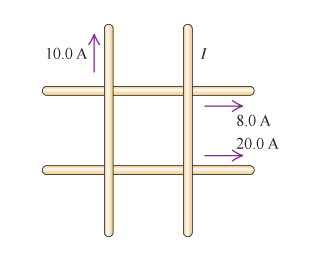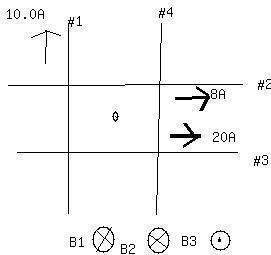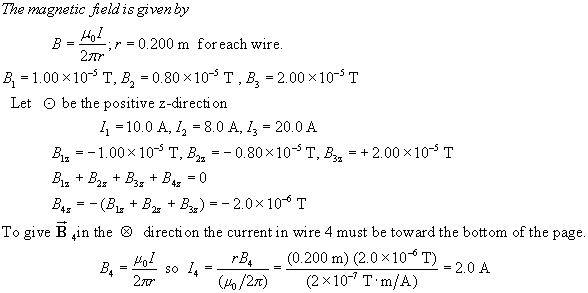# Four very long, current-carrying wires in the same plane intersect to form a square with side lengths of 45.0 cm, as shown in the figure blelow.Joni Kenny 2021-01-04 Answered

Four very long, current-carrying wires in the same plane intersect to form a square with side lengths of 45.0 cm, as shown in the figure blelow. The currents running through the wires are 8.0 A, 20.0 A, 10.0 A, and I.Part A
Find the magnitude of the current I that will make the magnetic field at the center of the square equal to zero.
Part B
What is the direction of the current I (up or down)?

You can still ask an expert for help

• Live experts 24/7
• Questions are typically answered in as fast as 30 minutes
• Personalized clear answers

Solve your problem for the price of one coffee

• Math expert for every subject
• Pay only if we can solve itstuth1

SolutionWe have step-by-step solutions for your answer!Jeffrey Jordon

(A)

Consider the direction ‘into the page’ to be negative and the direction ‘out of the page’ to be positive.

The distance of each wire from the center of the square is half the side of the square. Thus, the distance R can be written as follows:

$R=\frac{a}{2}$

Here, a is the side of the square.

Substitute 45.0 cm for a in the above expression.

= 22.5 cm

Use right-hand thumb rule to find the direction of the magnetic field at the center of the square due to the current 8 A.

Place the thumb of right-hand in the rightward direction in which the current is flowing. Curl the fingers around the wire to see that the magnetic field at the center of the square to be into the page. According to the assumed sign convention, the direction of magnetic field in this case should be negative.

The magnetic field at the center due to 8.0 A wire can be written as follows:

${B}_{8A}=-\frac{{\mu }_{o}I}{2\pi }$

Substitute 8.0 A for I in the above expression.

${B}_{8A}=-\frac{{\mu }_{o}\left(8A\right)}{2\pi R}$

The magnetic field at the center due to 20.0 A wire can be written as follows:

${B}_{20A}=\frac{{\mu }_{o}I}{2\pi R}$

Substitute 20.0 A for I in the above expression.

${B}_{20A}=\frac{{\mu }_{o}\left(20A\right)}{2\pi R}$

The magnetic field at the center due to 10.0 A wire can be written as follows:

${B}_{10A}=-\frac{{\mu }_{o}I}{2\pi R}$

Substitute 10.0 A for I in the above expression.

${B}_{10A}=-\frac{{\mu }_{o}\left(10A\right)}{2\pi R}$

Now, the magnetic field at the center of the square is 0. Thus, the sum of all the magnetic fields must be equal to zero.

${B}_{8.0\mathrm{A}}+{B}_{20.0\mathrm{A}}+{B}_{10.0\mathrm{A}}+B=0$

Here, B is the magnetic field in the 4th wire.

Substitute $-\frac{{\mu }_{\mathrm{o}}\left(8\mathrm{A}\right)}{2\pi R}$​ for ${B}_{8\mathrm{A}}$ , $\frac{{\mu }_{\mathrm{o}}\left(20\mathrm{A}\right)}{2\pi R}$ for ${B}_{20\mathrm{A}}$ , and $-\frac{{\mu }_{\mathrm{o}}\left(10\mathrm{A}\right)}{2\pi R}$ for ${B}_{10\mathrm{A}}$​ in the above expression.

$-\frac{{\mu }_{\mathrm{o}}\left(8\mathrm{A}\right)}{2\pi R}+\frac{{\mu }_{\mathrm{o}}\left(20\mathrm{A}\right)}{2\pi R}+\left(-\frac{{\mu }_{\mathrm{o}}\left(10\mathrm{A}\right)}{2\pi R}\right)+B=0$

$B=\frac{{\mu }_{\mathrm{o}}}{2\pi R}\left(20\mathrm{A}-8\mathrm{A}-10\mathrm{A}\right)$

$=-\frac{{\mu }_{\mathrm{o}}}{2\pi R}\left(2\mathrm{A}\right)$

The magnetic field due to the 4th wire is negative which means that the direction of magnetic field is ‘into the page’.

On comparing with the standard equation of magnetic field due to a current carrying wire, the current in the 4th wire is as follows:

$B=-\frac{{\mu }_{\mathrm{o}}I}{2\pi R}$

Substitute $-\frac{{\mu }_{\mathrm{o}}}{2\pi R}\left(2\mathrm{A}\right)$ for B in the above expression.

$-\frac{{\mu }_{\mathrm{o}}}{2\pi R}\left(2\mathrm{A}\right)=-\frac{{\mu }_{\mathrm{o}}I}{2\pi R}$

$I=2\mathrm{A}$

(B)

Due to wire with 8.0 A current, the magnetic field points ‘into the page’. This can be verified by right-hand thumb rule. Place the right hand in the direction of current 8.0 A. Curl the finger around it. The direction in which the fingers point at the center of the square gives the direction of the magnetic field due to this wire at the center. The direction comes out to be ‘into the page’.

Similarly, the direction of 20.0 A wire is ‘out of the page’.

Also, the direction of 10.0 A wire is ‘into the page’

Since, the magnetic fields due to 8.0 A and 10.0 A points ‘into the page’, they will add up.

This added quantity is still less than the magnetic field due to 20.0 A wire. Thus, there should be another 2.0 A current in the 4th wire, in a direction such that the remaining ‘out of the page’ magnetic field gets cancelled. For this, the magnetic field due to the 4th wire should point ‘into the page’.

Again, by right-hand thumb rule, curl the fingers around the 4th wire in such a way that the fingers goes into the page, the direction of the thumb will give the direction of the current in the 4th wire.

The direction of current comes out to be ‘downwards’.

We have step-by-step solutions for your answer!